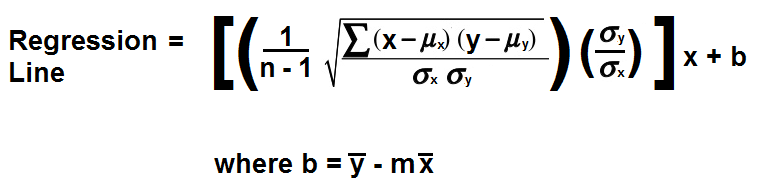﻿ Regression Line (Best Fit Line) Calculator ﻿# Regression Line (Best Fit Line) Calculator### Data Points

 X: Y: X: Y: X: Y: X: Y: X: Y: X: Y: X: Y: X: Y: X: Y: X: Y:

This Regression Line (Best Fit Line) Calculator calculates the best-fitting slope and best-fitting y-intercept for a linear line based on the given data points supplied.

So basically the gist of it is that there are data points.

A regression line is a line that tries its best to represent all of the data points as accurately as possible with a straight line. It strives to be the best fit line that represents the various data points.

A regression line can be calculated based off of the sample correlation coefficient. A sample correlation coefficient is a measure of the strength and direction of the linear relationship between 2 quantitative variables. If data points are perfectly linear, the sample correlation will either be 1 (for a line with a positive slope) or -1 (for a line with a negative slope). All values in between are not linear (with the exception of a vertical line which has a correlation coefficient of 0).

So based off of the sample correlation coefficient, we can determine the regression line, which represents the best-fitting (straight) line for a group of data points.

So the regression line is determined by the formula, y= mx+b, just like any line is.

However, how we determine the slope of the line is complex for a regression and is not simply rise/run, as the simple formula. To find the slope of a regression line (or best-fitting line), the formula is, slope, m= ((1/n-1)∑(x-μx) (y-μy)/σxσy)(σyx) Or if we take simplify by putting in r for the sample correlation coefficient, the formula is, slope, m= r(σyx)

So once you have computed the correlation coefficient, then calculating the best fit line is relatively simple.

We first calculate the slope through the formula, m= r(σyx)

Once we have done this, then we need to calculate the y-intercept. We do this by multiplying the slope by x. We then subtract this value from y. This is the y-intercept.

With the slope and y-intercept calculated, we then have our regression line.

### Example

Now that you know the steps to do, let's actually do an example.

So as an example, let's say we have a correlation coefficient of 0.98, x of 7, x of 12, σx of 4.58, and σy of 5.

So to find the slope, we use the formula, m= r(σyx)= 0.98(5/4.58)= 1.069

We then need to find the y-intercept. We multiply the slope by x, which is 1.069*7=7.489.

We then subtract this value from y, which is 12-7.489= 4.511

So our final regression line is, y= 1.069x + 4.511

To use this calculator, a user simply enters in the x and y value pairs. A user can enter anywhere from 3 to 10 (x,y) value pairs. After, the user clicks the 'Calculate' and the expected value will be calculated and automatically displayed.

Related Resources

﻿